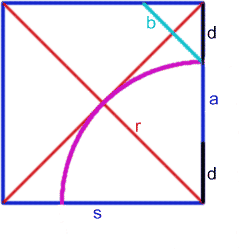#### You may also like### Diophantine N-tuples

Can you explain why a sequence of operations always gives you perfect squares?### DOTS Division

Take any pair of two digit numbers x=ab and y=cd where, without loss of generality, ab > cd . Form two 4 digit numbers r=abcd and s=cdab and calculate: {r^2 - s^2} /{x^2 - y^2}.### Sixational

The nth term of a sequence is given by the formula n^3 + 11n . Find the first four terms of the sequence given by this formula and the first term of the sequence which is bigger than one million. Prove that all terms of the sequence are divisible by 6.

# The Medieval Octagon

##### Age 14 to 16 Challenge Level:

Solution by: Andrew Ross from Dr. Challoner's Grammar SchoolCall the side length of the square $s$. Use the diagram for other lengths.

The octagon has side lengths $a$ and $b$.

By Pythagoras' Theorem: $$r = {s\sqrt 2\over 2}$$ Because $s = r + d$ so $$d = s(1 - {\sqrt 2 \over 2}).$$ One side of the octagon is given by: $$a = s - 2d = s - 2s(1 - {\sqrt 2 \over 2}) = s(\sqrt 2 - 1).$$ By Pythagoras' Theorem $b^2 = 2 d^2$ so $b = d \sqrt 2$. Hence $$b = s\sqrt 2 (1 - {\sqrt 2 \over 2}) = s(\sqrt 2 - 1) = a.$$ The octagon has rotational symmetry of order 4 as it was constructed from a square and all the sides are the same length, so it is a regular octagon.